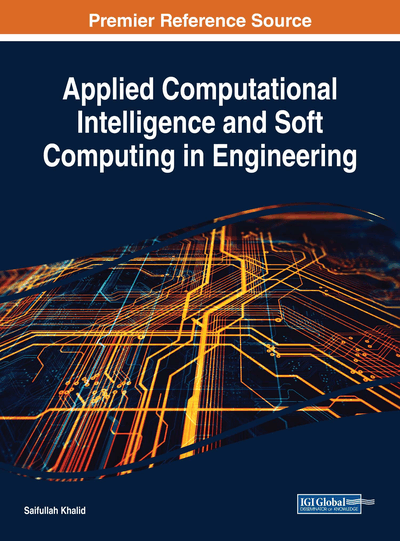# Metaheuristic- and Statistical-Based Sampling in Optimization

Yoel Tenne (Ariel University, Israel)
DOI: 10.4018/978-1-5225-3129-6.ch004
Available
\$37.50
No Current Special Offers

## Abstract

Modern engineering often uses computer simulations as a partial substitute to real-world experiments. As such simulations are often computationally intensive, metamodels, which are numerical approximations of the simulation, are often used. Optimization frameworks which use metamodels require an initial sample of points to initiate the main optimization process. Two main approaches for generating the initial sample are the ‘design of experiments' method which is statistically based, and the more recent metaheuristic-based sampling which uses a metaheuristic or a computational intelligence algorithm. Since the initial sample can have a strong impact on the overall optimization search and since the two sampling approaches operate based only widely different mechanisms this study analyzes the impact of these two approaches on the overall search effectiveness in an extensive set of numerical experiments which covers a wide variety of scenarios. A detailed analysis is then presented which highlights which method was the most beneficial to the search depending on the problem settings.
Chapter Preview
Top

## Introduction

Computer simulations, also termed in the literature as computer experiments, are used in engineering and science as a partial replacement to actual laboratory experiments. In this setup, candidate designs are represented as a vector of design variables and are sent to the computer simulation to evaluate how well they satisfy the design goals. This simulation-driven process results in an optimization problem with three distinct characteristics (Tenne & Goh, 2010a):

• The numerical simulation is often intricate, for example, involving the iterative solution of large sets of partial differential equations as commonly done in computational fluid mechanics (CFD) or computational structural mechanics (CSM). As such, there is no analytic relation which explains how an input vector is mapped to an output value, and thus the simulation is referred to in the literature as a black-box function. The lack of an analytic expression adds an optimization difficulty.

• Typically each run of the numerical simulation is computationally intensive, namely, requiring large computational resources, and hence only a small number of candidate designs can be directly evaluated with the simulation.

• The aforementioned ‘black-box’ function may have complicated features such as multiple local optima or discontinuities which are an outcome of either the underlying physics of engineering problem or result from the numerical simulation process itself. Regardless, such features render the task of locating an optimum even more difficult.

To circumvent these challenges optimization frameworks often use metamodels (also termed in the literature as surrogates or response surfaces), which are numerical approximations of the inputs-output mapping of the simulation but which are significantly cheaper to evaluate in terms of computational cost (Tenne & Goh, 2010a). Optimization frameworks which rely on such metamodels require an initial sample of vectors to generate a preliminary metamodel as a precursor step to the main optimization process. It follows that the initial sample can strongly impact the overall effectiveness of the optimization, which in turn motivates an analysis of this linkage.

Approaches for generating the initial sample include the statistical design of experiments (DOE) methodology in which the vectors are a-priori sampled to satisfy some optimality criterion (Chen et al., 2006; Queipo et al., 2005), and the more recent metahueristic based sampling (MHS) in which a metaheuristic optimization algorithm is used for a short duration and the vectors it generated are then used as the initial sample (Büche et al., 2005; Liang et al., 2000; R. Jin et al., 2005). Existing studies from the domain of metamodel-assisted optimization have focused mainly on the DOE methods, for example, as discussed by Queipo et al. (2005);

Chen et al. (2006); Sóbester et al. (2005); Forrester & Keane (2008); Wang & Shan (2007). However, the MHS approaches have received much less attention, in particular when their merits and demerits are compared to DOE methods. Accordingly, this chapter addresses this open question and extensively analyzes the impact of DOE and MHS sampling methods in the context of metamodel-assisted optimization. The analysis is performed through an extensive set of numerical experiments in which representative methods from each category were used in a wide range of optimization scenarios. Based on the results of these experiments a detailed analysis is presented as to which approach was preferable based on the settings of the problem which was being solved.

The remainder of this chapter is organized as follows: Section 2 provides the background information, Section 3 describes in detail the numerical experiments performed, Section 4 provides an extensive analysis and discussion, and lastly Section 5 concludes this chapter.

## Complete Chapter List

Search this Book:
Reset